The ENTROPY Procedure (Experimental)

Statistical Tests

Subsections:

Since the GME estimates have been shown to be asymptotically normally distributed, the classical Wald, Lagrange multiplier, and likelihood ratio statistics can be used for testing linear restrictions on the parameters.

Wald Tests

Let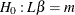, where
is a set of linearly independent combinations of the elements of. Then under the null hypothesis, the Wald test statistic,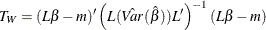has a centrallimiting distribution with degrees of freedom equal to the rank of.

Pseudo-Likelihood Ratio Tests

Using the conditionally maximized entropy function as a pseudo-likelihood,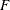, Mittelhammer and Cardell (2000) state that: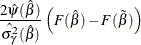has the limiting distribution of the Wald statistic when testing the same hypothesis. Note that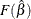and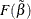are the maximum values of the entropy objective function over the full and restricted parameter spaces, respectively.

Lagrange Multiplier Tests

Again using the GME function as a pseudo-likelihood, Mittelhammer and Cardell (2000) define the Lagrange multiplier statistic as: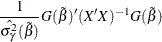where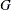is the gradient of, which is being evaluated at the optimum point for the restricted parameters. This test statistic shares the same limiting distribution as the Wald and pseudo-likelihood ratio tests.